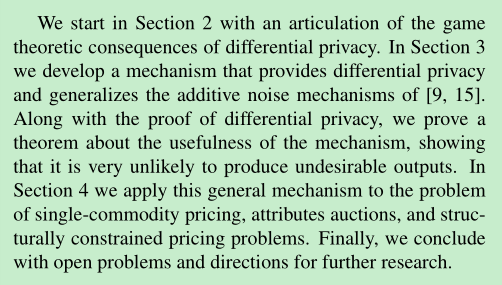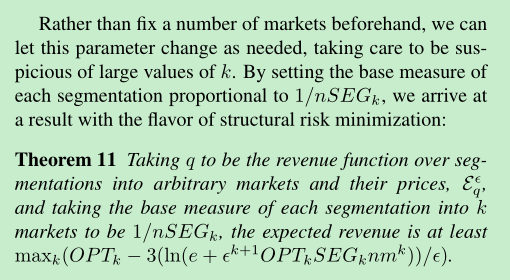Frank McSherry, Kunal Talwar. Mechanism design via differential privacy. FOCS 2007.

Paper Outline：• Approximate Truthfulness

• Collusion Resistance

• Composability

## A General dp Mechanism

• domain: $\mathcal{D}$，range: $\mathcal{R}$
• query function：$q:\mathcal{D}^n\times \mathcal{R}\to\mathbb{R}$，是对 (input, output) 二元组的估值，$q$ 越大，二者越匹配.

### 准确性

• $\mu(A)$$A\subseteq\mathcal{R}$ 的测度，且 $\mu(\mathcal{R})=1$
• $p(A)$$\mathcal{E}_q^\epsilon(d)$ 所定义的测度（同样也进行正则化）；
• $OPT=\max_r q(d,r)$.

## Applications to Pricing and Auctions

• 每个参与者都有一个需求函数 $b_i:[0,1]\to\mathbb{R}^+$，表示在定价为 $p\in[0,1]$ 时该参与者对物品的需求量；
• 为符合常理，每一个 $b_i$ 都是不增的，且 $\forall i,p$$pb_i(p)\leqslant 1$
• $\forall$ 价格 $p$，我们可以卖掉 $\sum_i b_i(p)$ 件商品，取总收益为 $q(b,p)=p\sum_i b_i(p)$.

General mechanism 还可以推广到 attribute auctions.

• $OPT_k$ 表示分割为 $k$ 部分的市场的最大收入；
• $SEG_k$ 表示 $n$ 个竞拍者进入 $k$ 个市场时被允许划分的部分数；
• 有结果表明 $SEG_k\leqslant n^{kVC}$.• 每个 $i$ 对物品 $j\in[k]$ 有一个需求函数 $b_i^j:[0,1]\to\mathbb{R}^+$
• 须对 $\forall i,j,p$ 确保 $pb_i^j(p)\leqslant 1$.
• $\mathcal{R}=[k]\times\mathbb{R}$
• 对物品 $j$，价格为 $p$，可以卖掉 $\sum_i b_i^j(p)$ 件，得到 $q(b,(j,p))=p\sum_i b_i^j(p)$ 的收入.